# Focal Length Calculator (Thick Lens)

This is an online calculator to find the focal length of a thick lens. Just enter the radius of curvature, refractive index, and thickness to get the nominal power, effective power, and focal length value.

• mm
• mm
• mm

### Result

• Nominal Power
• Effective Power
• Effective Focal Length
mmThick lens is a physically large lens whose spherical surfaces are separated by a distance. In other words, it is a lens whose thickness cannot be neglected when compared to its focal length.

Focal length is the distance between the focal point and the optical center of a lens. It is the measure of the ability of a lens to converge or diverge a ray of light. The plane perpendicular to the principal axis of the lens and passing through its focal point is known as the focal plane.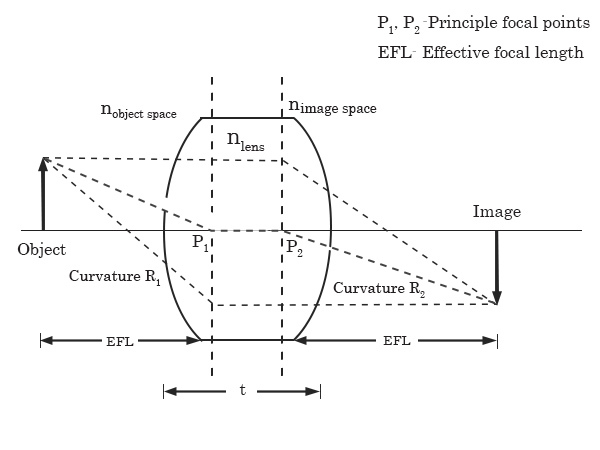Focal Point is the point to which a set of rays parallel to the principal axis is set to converge (in the case of a convex lens) or appear to diverge (in the case of a concave lens).

Optical Center is a point on the principal axis for which the rays passing through it are not deviated by the lens. Any ray passing through the optic center emerges in a direction parallel to the incident ray, i.e. the ray emerges undeviated.

Principle Axis is the line joining the centers of curvature of the two curved surfaces of a lens.

A lens has two curved surfaces and each surface has a curvature.

Radius of Curvature of a lens is the radius of that circle of which the curvatures of the lens is a part.

Power of a lens is the measure of its ability to produce convergence of a parallel beam of light. A convex lens has a converging effect and thus its power is taken as positive, but a concave lens produces divergence and so its power is taken as negative. The unit in which the power of a lens is measured is called Dioptre. Mathematically,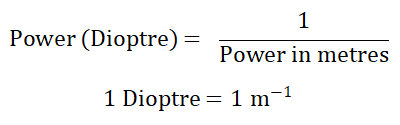In the case of a thick lens, the optical power of the surfaces depends on the refractive index of the lens. Thus the power output of both surfaces will be different.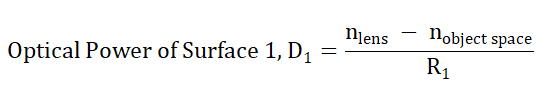Where nlens is the refractive index of the lens, nobject space is the refractive index of the medium in which the object is kept and R1 is the radius of curvature of surface 1.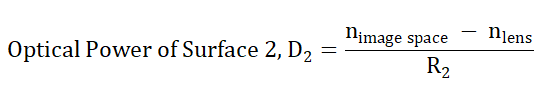nimage space is the refractive index of the medium in which the image is formed, and R2 is the radius of curvature of surface 2.

Nominal Power of a lens is algebraically the sum of optical powers of each surface. It is denoted as 'DNP'.

Where, DNP = D1 + D2

Since the thickness is non-negligible, the Effective Power is not just simply the sum of the individual powers, but it depends on the thickness of the lens. The equation for the effective power of a lens is given by the Gullstrand Equation as,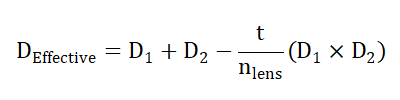where 't' is the thickness of the lens.

In such a lens where the power depends on the thickness of the lens, the effective focal length cannot be taken as the sum of individual foci of the two surfaces. So, we define Effective Focal Length as the reciprocal of the effective power of the lens, showing the thickness dependence of the lens.